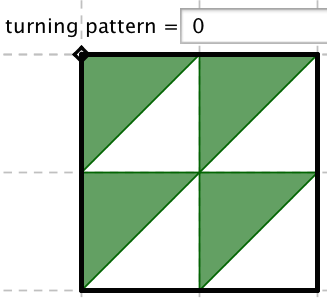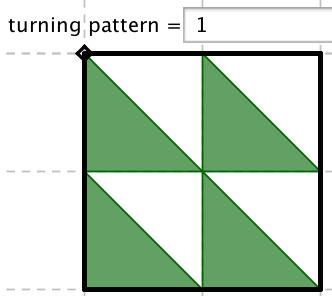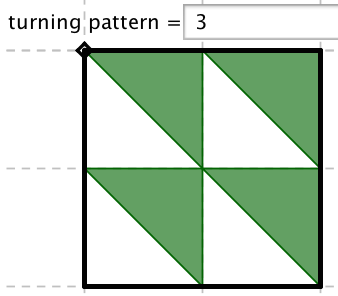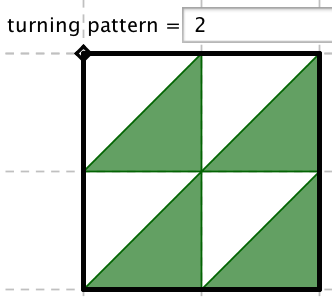## Thursday, May 1, 2014

### Truchet

Last month, the always charming Math Munch had a post on Truchet squares. In their simplest form, rectangular arrays of squares with one right isosceles half colored in. I'd kept them in the back of my head to play with in GeoGebra, then yesterday had a freeish day and a conversation with a quilter friend that brought them back to mind.

The first part of this post is making the GeoGebra, the second is playing around with the new tool. (Here's the sketch on GeoGebraTube if you'd rather play than read.)

GeoGebra
The first goal was to make a random Truchet square. I knew I'd want to have size choices, so I put in a slider for that. I made a tool to make a right isosceles triangle from a center point and one corner of a square. I thought I could make an array of square center points, and then rotate corners around.  Nested sequences are good for arrays of points, like:
list1=Sequence[Sequence[A + (2i, -2 j), i, 0, n - 1], j, 0, n - 1]
But that will make you a list of lists of points. Sometimes what you want, but not here. Easy to fix, though, with:
list4=Join[list1]

I thought I could make my random corners with a sequence command, like
Sequence[Element[list4,i]+(RandomElement[{-1,1}], RandomElement[{-1,1}]), i, 1, length[list4]]
But it turns out that will only call the RandomElement command once! Not so random looking if they all have the same value. The work around I found in the GeoGebra Forum. Make a list of random values directly, then use that list. So I made two lists of random 1 or -1, then
Sequence[Element[list4, i] + (Element[listx, i], Element[listy, i]), i, 1, Length[list4]]

The second option is just an array of triangles fixed to a square array of points. Part of the reason the grid is turned on is because that helps those snap to the corners. Unfotunately, I don't know a way to make those kind of things in a list; or rather, I can, but the resulting points aren't individually selectable. So I just made the array and circles around them by sequence, then the TruchetTri tool I had made to make the triangles.

The third option was the real goal for me. I wanted to make a way to put in a rule, then have the Truchet pattern show the rule. Essentially, you want a sequence with values 0 to 3 to show which position the Truchet triangle is in.So I thought of it as rotating the upper right corner around the center by a multiple of 90°. Then it would naturally be modular. So any function that sends integers to integers would work! That sounded fun to me. The final command was:
Sequence[Rotate[Element[list4, i] + (1, 1), (turn(i) 90)°, Element[list4, i]], i, 1, Length[list4]]
where turn(x) is the function that you input.

Time to Play
So what can you make?

The random can be fun:It's amazing the patterns your mind will make up and see. Lots of these shapes do show up in the patterned squares that you make.

The design your own can be interesting. I'd imagine students making many patterns or symmetrical designs on their own. Hopefully that would lead to some graph paper doodling.

But as I said, the rules were what I wanted to play with. Polynomials with integer coefficients are integer to integer maps. Here's turn(x)=x and turn(x)= \( x^3 \).The different grid sizes pay off here, as you can get different effects depending on how often you wrap around. Here's turn(x)= \( x^4 +x \) in a 5x5 and 6x6.